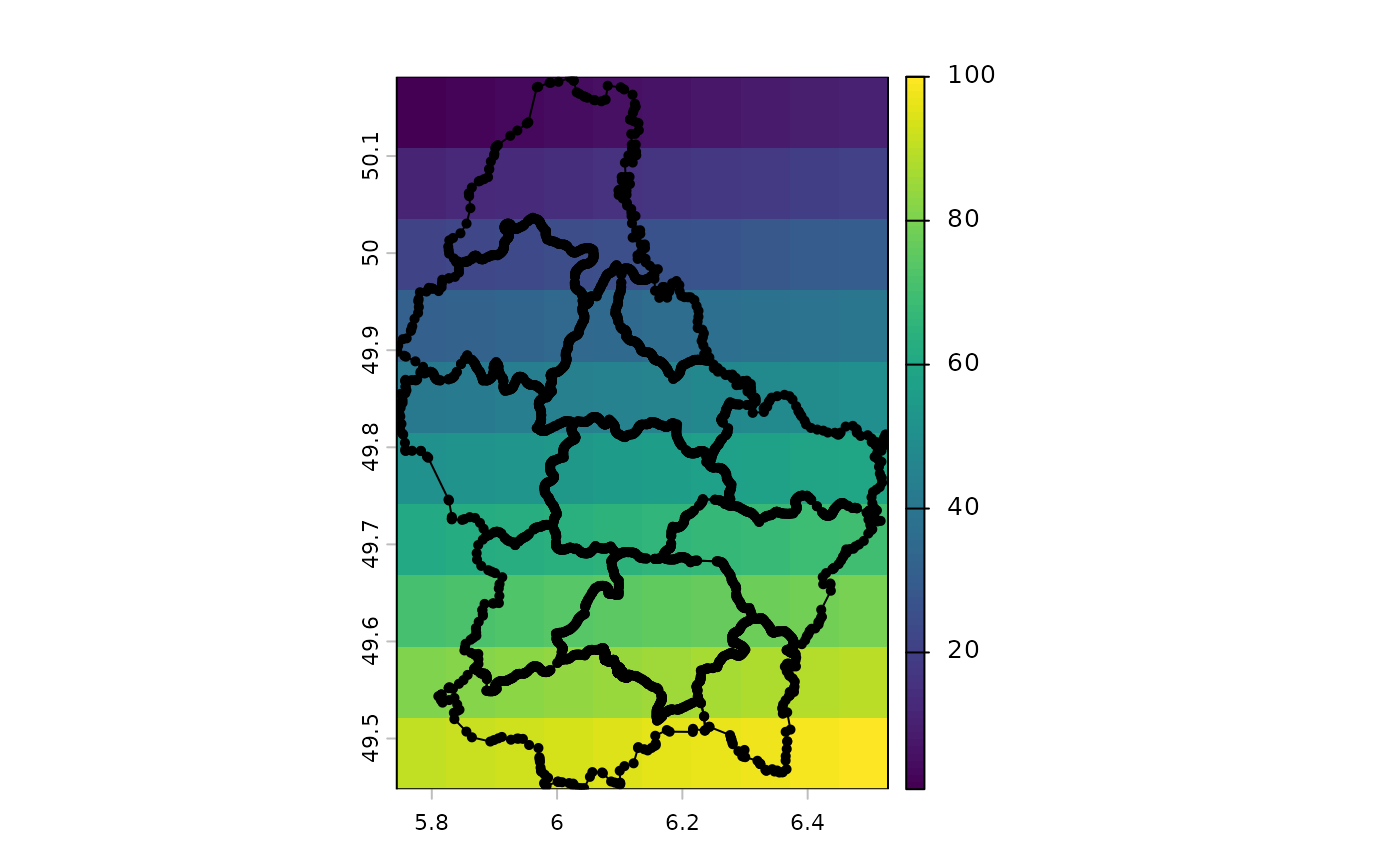Add a vector geometries to a plot (map) with points, lines, or polys.

These are simpler alternatives for plot(x, add=TRUE)

These methods also work for a small(!) SpatRaster. Only cells that are not NA in the first layer are used.

# S4 method for SpatVector
points(x, col, cex=0.7, pch=16, alpha=1, ...)

# S4 method for SpatVector
lines(x, y=NULL, col, lwd=1, lty=1, arrows=FALSE, alpha=1, ...)

# S4 method for SpatVector
polys(x, col, border="black", lwd=1, lty=1, alpha=1, ...)

# S4 method for SpatRaster
points(x, ...)

# S4 method for SpatRaster
lines(x, mx=10000, ...)

# S4 method for SpatRaster
polys(x, mx=10000, dissolve=TRUE, ...)

# S4 method for SpatExtent
points(x, col="black", alpha=1, ...)

# S4 method for SpatExtent
lines(x, col="black", alpha=1, ...)

# S4 method for SpatExtent
polys(x, col, alpha=1, ...)

## Arguments

x

SpatVector or SpatExtent

y

missing or SpatVector. If both x and y have point geometry and the same number of rows, lines are drawn between pairs of points

col

character. Colors

border

character. color(s) of the polygon borders. Use NULL or NA to not draw a border

cex

numeric. point size magnifier. See par

pch

positive integer, point type. See points. On some (linux) devices, the default symbol "16" is a not a very smooth circle. You can use "20" instead (it takes a bit longer to draw) or "1" for an open circle

alpha

number between 0 and 1 to set transparency

lwd

numeric, line-width. See par

lty

positive integer, line type. See par

arrows

logical. If TRUE and y is a SpatVector, arrows are drawn intead of lines. See ?arrows for additional arguments

mx

postive number. If the number of cells of SpatRaster x is higher, the method will fail with an error message

dissolve

logical. Should boundaries between cells with the same value be removed?

...

additional graphical arguments such as lwd, cex and pch

## Examples

f <- system.file("ex/lux.shp", package="terra")
v <- vect(f)

r <- rast(v)
values(r) <- 1:ncell(r)
plot(r)
lines(v)
points(v)